Transformations

Transformations

 Site: TBAISD Moodle Course: Michigan Algebra I Preview 2012 Book: Transformations Printed by: Guest user Date: Saturday, December 14, 2019, 08:38 AM

Transformation

Function notation for the parent function of a quadratic is f(x) = x2. A function transformation takes the basic function f(x) and then "transforms" it, so that the graph will become more wide or narrow, reflect across the x-axis, or translate up, down, left or right.

To translate the graph of a quadratic function, you can use the vertex form, y = a(x - h) 2 + k.

• The h-value will translate the graph to the right when it is negative and to the left when it is positive.
• The k-value will translate the graph up when it is positive and down when it is negative.

Example The equation,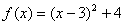, represents what translation of the parent function,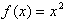?

Since the h -value is negative, the translation will be 3 units to the right. Since the k -value is positive, the translation will be up 4 units.

Listen

Up or Down

To understand the effects of k, compare the functions y = x2 and y = x2 + 3. The table below shows the pattern of values.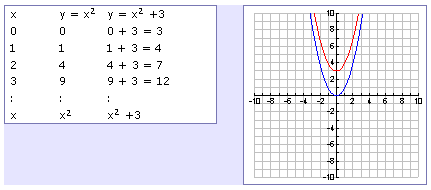By looking at both the table of values and the graph, k produces a vertical shift; in this case, k = 3 shifts the parabola up 3 units.

In function notation, to move a function up, add outside the function. The function f(x) + c is f(x) moved up c units. Moving the function down works the same way, the function f(x) - c is f(x) moved down c units.

Listen

Left or Right

To understand the effects of h, compare the functions y = x2 and y = (x + 3)2. The table below shows the patterns of values.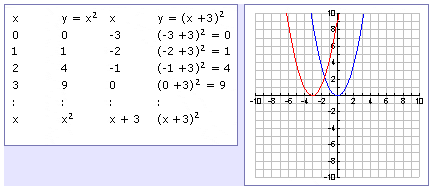By looking at both the table of values and the graph, it appears as though h produces a horizontal shift; in this case, h = -3 shifts the parabola 3 units to the left. Expressing the equation in vertex form, y = (x + 3)2 = (x - (-3))2. In general, the graph is shifted h units to the right if h is positive, and h units to the left if h is negative.

In function notation, to shift a function left, add inside the function's argument: f(x + b) shifts f(x) b units to the left. Shifting to the right works the same way, f(x - b) shifts f(x) b units to the right.

*Note: Remember a sign on the inside of the function does the opposite as a sign on the outside of the function. This is because in order to turn f(x + b) into f(x), x = -3 which graphs to the left.

Effects of "a"

When the a-value is greater than one, the parabola becomes narrower. When the a-value is less than one, the parabola becomes wider. When the a-value is positive, the parabola will open upward; when it is negative the parabola will open downward.
Example
The graph below represents the function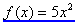in blue and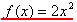in red. Since both a -values are positive, the parabolas open up. Since 5 > 2, the blue parabola will be narrower than the red parabola.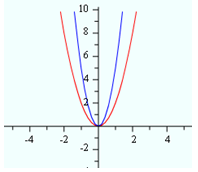Listen

Reflecting Over the x-axis

Another effect of "a" is to reflect the graph across the x-axis. When the parent function f(x) = x2 has an a-value that is less than 0, the graph reflects across the x-axis before it is transformed. The graph below represents the function f(x) = -x2.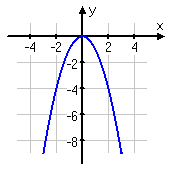In function notation, this reflection is represented by a negative outside the function: -f(x). If the negative is inside the function notation, there is a reflection across the y -axis. Since a parabola is already symmetric about the y -axis, this transformation does not actually change the look of the parabola.

Listen

Summary of the Rules

Transformations of any family of functions follow these rules:

• f(x) + c is f(x) translated upward c units
• f(x) - c is f(x) translated downward c units
• f(x + b) is f(x) translated left b units
• f(x - b) is f(x) translated right b units
• -f(x) is f(x) reflected about the x-axis
• f(-x) is f(x) reflected about the y-axis
• a•f(x) stretches the graph vertically if a > 1
• a•f(x) shrinks the graph vertically if 0 < a < 1
Listen

Equations

When writing the equation of a transformation, determine how the graph has moved in the coordinate plane compared to the parent function and then use the rules to write an equation.

Example 1 The graph of y = x2 is transformed to produce the new red graph. What's the new equation?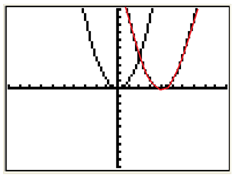Step 1. Determine the transformation of the graph.

This graph has been translated 4 units right.

Step 2. Use the transformation rules to write an equation.

f(x - b) translates the graph to the right.

The equation will be:
y
= (x - 4) 2

Interactive Activity

Transformations of Parabolas

Interactive Practice

For practice on transforming functions, select the following link:

Transforming Functions

Guided Practice

To solidify your understanding of transforming quadratic functions, visit the following link to Holt, Rinehart and Winston Homework Help Online. It provides examples, video tutorials and interactive practice with answers available. The Practice and Problem Solving section has two parts. The first part offers practice with a complete video explanation for the type of problem with just a click of the video icon. The second part offers practice with the solution for each problem only a click of the light bulb away.

Guided Practice

Practice

Transformations of Parabolas Worksheet